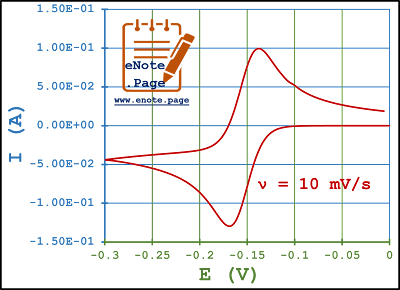## Posts

Showing posts with the label Specific Capacitance of a Supercapacitor from Cyclic Voltammetry data

### Specific Capacitance of a Supercapacitor from Cyclic Voltammetry dataFormula   C s - Specific capacitance (F/g) = to be calculated. A - Area under the I vs. E curve (AV) = 0.0154 m - Mass of the electrode material (g) = 20 mg = 0.02 g ν - Scan rate (V/s) = 10 mV/s = 0.01 V/s dV - Potential window (difference in potential) = 0.3 (= 0.0 V (-0.3 V))   Area (A) under the curve can be calculated using Origin program , whereas 'dV' is the difference in the potential. Refer the video tutorial for the calculations of 'A' and 'dV'. https://youtu.be/AzfnyTgADh0   By, Dr. M Kanagasabapathy Associate Professor Department of Chemistry Rajapalayam Rajus College Madurai Kamaraj University Rajapalayam 626117 (TN)